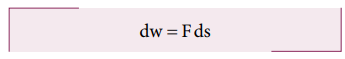Home | | Physics 11th std | Work done by Torque

# Work done by Torque

Let us consider a rigid body rotating about a fixed axis.

Work done by Torque

Let us consider a rigid body rotating about a fixed axis. Figure 5.29 shows a point P on the body rotating about an axis perpendicular to the plane of the page. A tangential force F is applied on the body.

It produces a small displacement ds on the body. The work done (dw) by the force is,dw = Fds

As the distance ds, the angle of rotation dθ and radius r are related by the expression,

ds = rdθ

The expression for work done now becomes,

dw = F ds; dw = F r dθ

The term (Fr) is the torque τ produced by the force on the body.

dw = τdθ        (5.51)This expression gives the work done by the external torque τ, which acts on the body rotating about a fixed axis through an angle dθ.

The corresponding expression for work done in translational motion is,

dw = FdsStudy Material, Lecturing Notes, Assignment, Reference, Wiki description explanation, brief detail
11th Physics : UNIT 5 : Motion of System of Particles and Rigid Bodies : Work done by Torque |